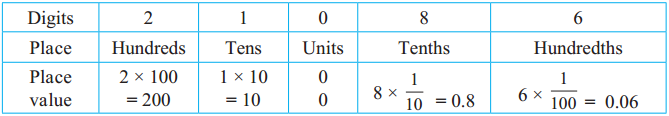# Maharashtra Board Class 5 Maths Solutions Chapter 9 Decimal Fractions Problem Set 37

Balbharti Maharashtra Board Class 5 Maths Solutions Chapter 9 Decimal Fractions Problem Set 37 Textbook Exercise Important Questions and Answers.

## Maharashtra State Board Class 5 Maths Solutions Chapter 9 Decimal Fractions Problem Set 37

Write the following mixed fractions in decimal form and read them aloud.

$$\text { (1) } 9 \frac{1}{10}$$
9.1, Nine point one.$$\text { (2) } 9 \frac{1}{100}$$
9.01, Nine point zero one.

$$\text { (3) } 4 \frac{53}{100}$$
4.53, Four point five three.

$$\text { (4) } \frac{78}{100}$$
0.78, Zero point seven eight.

$$\text { (5) } \frac{5}{100}$$
0.05, Zero point zero five.

$$\text { (6) } \frac{5}{10}$$
0.5, Zero point five.$$\text { (7) } \frac{2}{10}$$
0.2, Zero point two.

$$\text { (8) } \frac{20}{100}$$
0.20, Zero point two zero.

Place value of the digits in decimal fractions

We can determine the place value of the digits in decimal fractions in the same way that we determine the place values of digits in whole numbers.

Example (1)
In 73.82, the place value of 7 is 7 × 10 = 70, and of 3, it is 3 × 1 = 3.
Similarly, the place value of 8 is 8 × $$\frac{1}{10}=\frac{8}{10}$$ = 0.8 and the place value of 2 is 2 × $$\frac{1}{100}=\frac{2}{100}$$ = 0.02

Example (2)
Place values of the digits in 210.86.$$\text { (1) } 3 \frac{3}{100}$$
3.03, Three point zero three.

$$\text { (2) } 3 \frac{33}{100}$$
3.33, Three point three three.

$$\text { (3) } 30 \frac{41}{100}$$
30.41, Thirty point four one.

$$\text { (4) } 11 \frac{11}{100}$$
11.11, Eleven point one one.Q.3. Write the following numbers using the decimal point:
(1) Sixty-eight point seven six .
68.76

(2) Nine point five zero one
9.501

(3) Eighty-four point zero three.